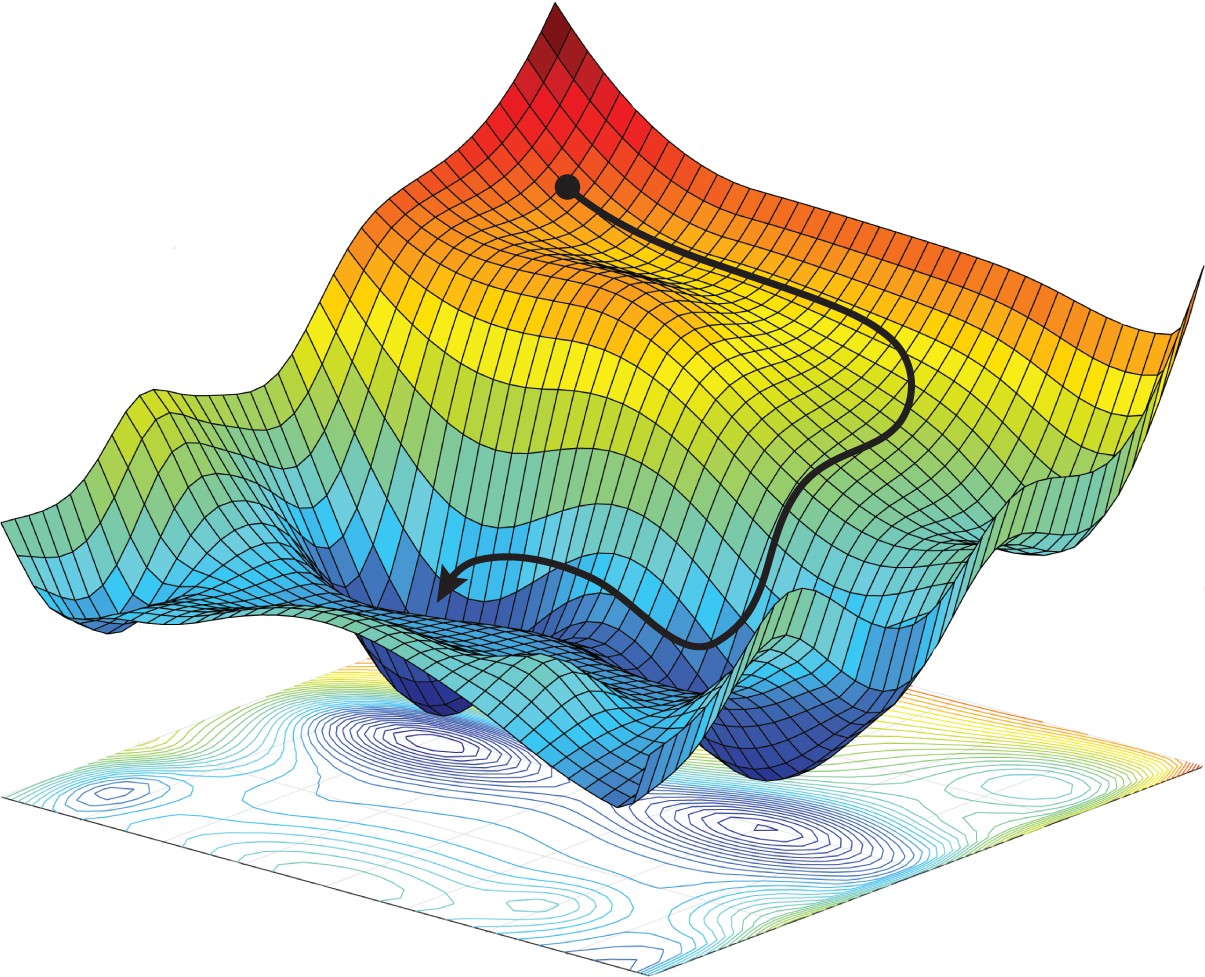## Inverse Problems and Optimization

#### Review the fundamentals and the main issues of inverse problems and focus on state‐of‐the‐art and recently introduced inversion procedures and algorithms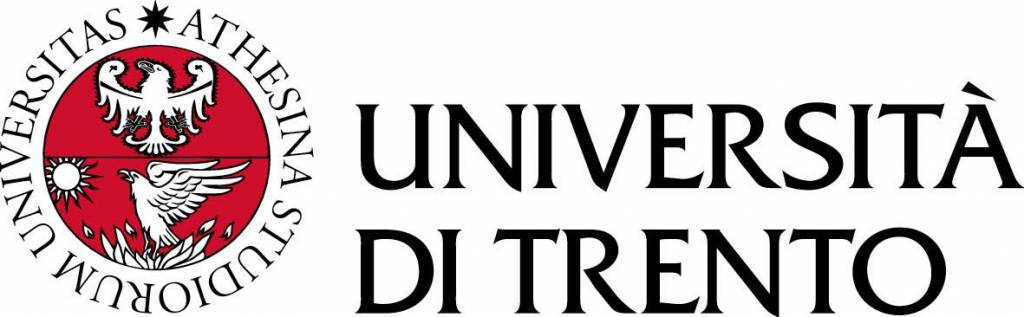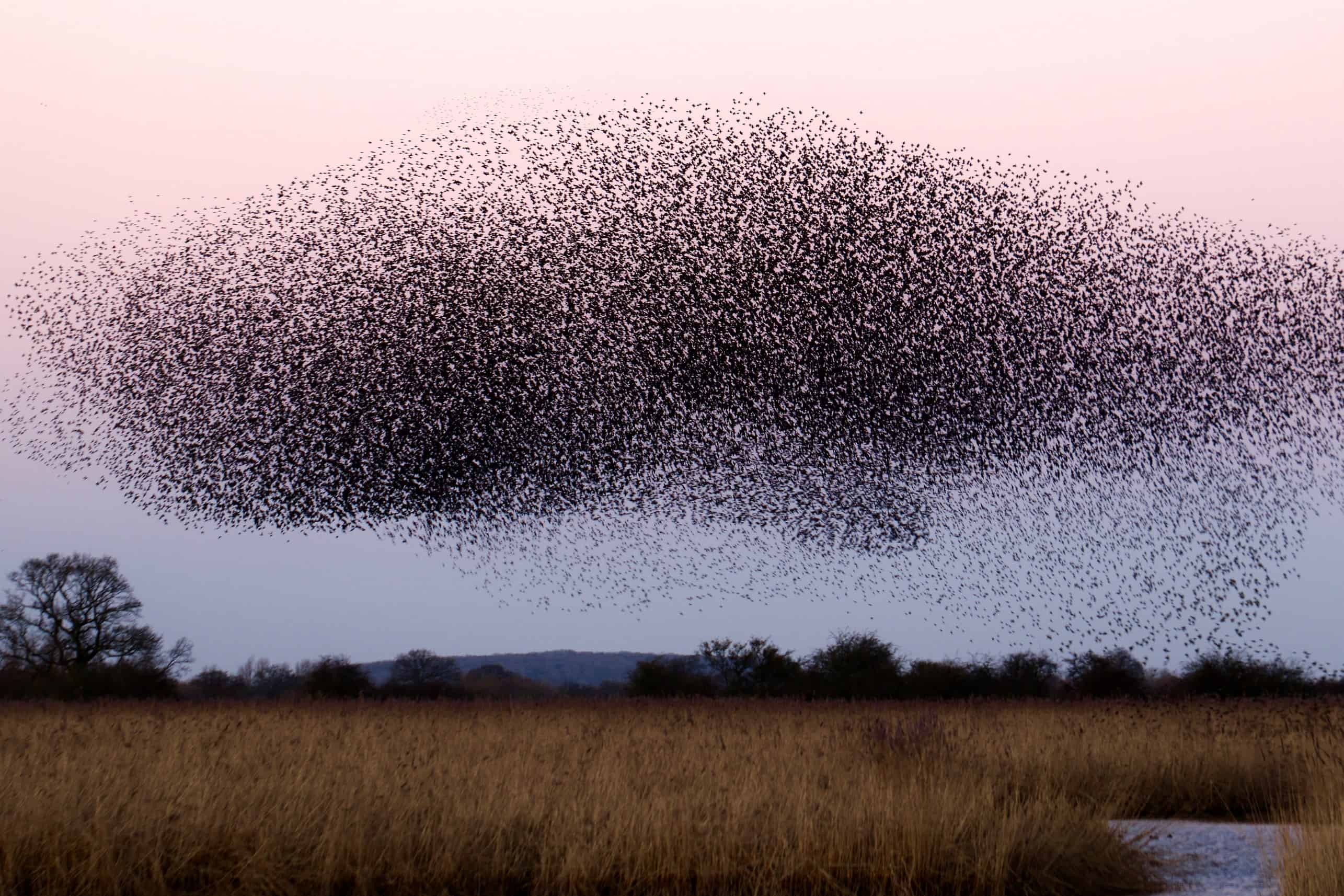##### The course will review fundamentals and main issues of inverse problems then focusing on classical/state-of-the-art and recently introduced inverse solution procedures and algorithms and their use through a professional optimization framework. Applicative examples including exercises will corroborate the theoretical concepts. The first part is devoted to introduce the students to the concept of inverse problems and to their general formulation. The second part reviews the mathematical issues of inverse problems and the third part is aimed to teach the strategies to overcome such drawbacks. The last part presents how to deal with the solution of inverse problems through optimization methodologies. In short, the course attendees will:
1. learn about the basics of inverse problems;
2. enhance their background on inverse problems;
3. know about state-of-the art inversion algorithms;
5. use of professional optimization tools for the solution of inverse problems.

### Course Program

##### Part 1 : INTRODUCTION AND BASICS

• Motivations (methodological, applicative); synthesis and design problems as inverse problems;
• Formulation of an inverse problem and numerical techniques for dealing with their resolution.

##### Part 2 : INVERSION PROBLEMS: ISSUES AND DRAWBACKS

• Ill-posedness and non-linearity. On the role of information in inverse problems;
• Ill-posedness: the need for regularization;
• non-linearity: physical meaning, degree of non linearity, the role of a-priori and of the amount of available information.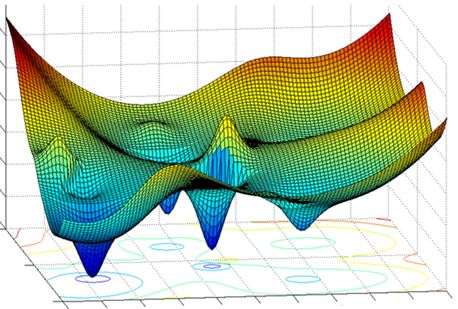##### Part 3 : SOLVING INVERSION PROBLEMS THROUGH OPTIMIZATION

• Solution of the inverse problems as minimization/maximization of a cost-function/functional;
• Taxonomy of optimization approaches and description: single agent and multiple agents; deterministic and stochastic optimization.

##### Part 4: DETERMINISTIC ALGORITHMS

• Deterministic optimization: Steepest Descent Method; Conjugate-Gradient Method;
• EXERCISE: Use of the Steepest Descent Method for the solution of inverse problems;
• STUDENT PROJECT (A): supervised implementation of the Conjugate-Gradient Method.

##### Part 5: STOCHASTIC ALGORITHMS

• Stochastic ‘nature-inspired’ optimization: competitive optimization (Genetic Algorithm, Differential Evolution); cooperative optimization (Particle Swarm Optimization, Ant Colony Optimization).
• EXERCISE: Use of the Differential Evolution for the solution of inverse problems;
• STUDENT PROJECT (B): supervised use of professional optimization tools described in the context of the research by the ELEDIA Research Center on the solution of inverse problems through optimization. Elaboration and critical analysis of the results and preparation of appropriate scientific report.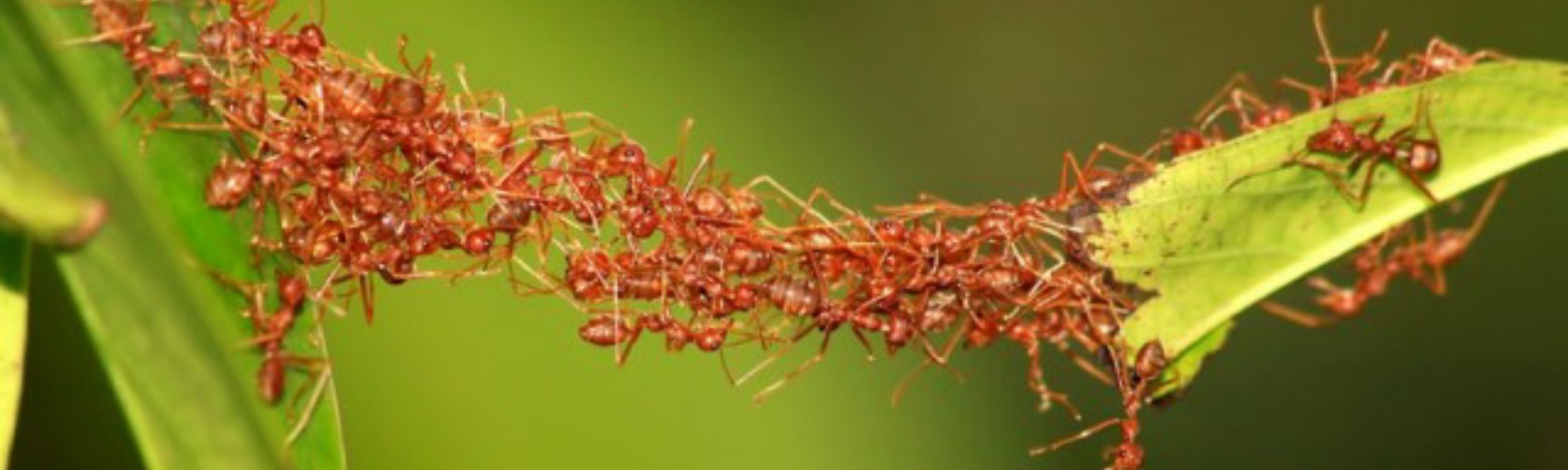### Teaching Modalities

Theoretical lessons. Seminars with slides. Software exercises.

### Prerequisites

Basic courses of Mathematical Analysis.

### Textbooks and References

• F. D. Moura Neto, A. J. da Silva Neto, “An Introduction to Inverse Problems with Applications”. Springer, 2013.
• A. Tarantola, “Inverse Problem Theory and Methods for Model Parameter Estimation”. SIAM, 2005.
• R. C. Aster, B. Borchers, and C. H. Thurber, “Parameter Estimation and Inverse Problems”. Elsevier, 2013.
• M. Bertero and P. Boccacci, “Introduction to Inverse Problems in Imaging”. IoP Press, 1998.
• F. D. Moura Neto and A. J. da Silva Neto, "An Introduction to Inverse Problems with Applications," Ed: Spring, 2013.
• D. J. Rader, “Deterministic Operations Research: Models and Methods in Linear Optimization”. John Wiley & Sons, 2010.
• S. Boyd and L. Vandenberghe, “Convex Optimization”. Cambridge University Press, 2004.
• D. Simon, “Evolutionary Optimization Algorithms”. John Wiley & Sons, 2013.
• K. Deb, “Multi‐Objective Optimization Using Evolutionary Algorithms”. John Wiley & Sons, 2001.
• R. Battiti and M. Brunato, “The LION way. Machine Learning plus Intelligent Optimization”. LIONLab, 2015.
• P. Rocca, M. Benedetti, M. Donelli, D. Franceschini, and A. Massa, "Evolutionary optimization as applied to inverse scattering problems," Inverse Problems, Invited Topical Review Paper ‐ 25th Year Special Issue of Inverse Problems, vol. 24, pp. 1‐41, 2009.

• Teacher:
• Syllabus:
• Material:
• Course:
• Institution:
• Language:
English